Anúncio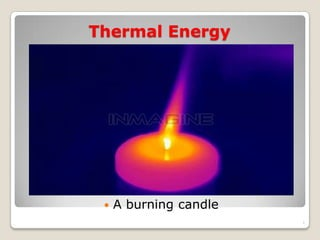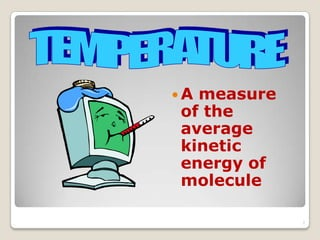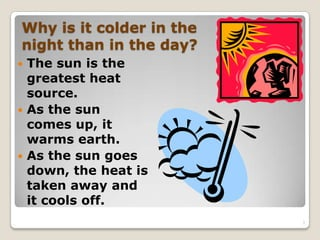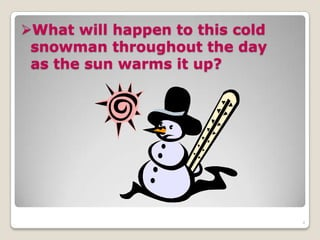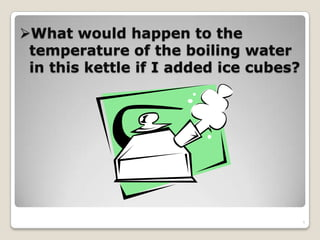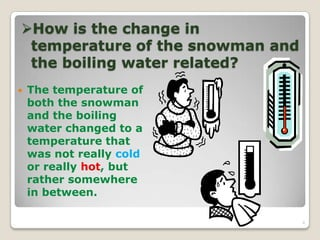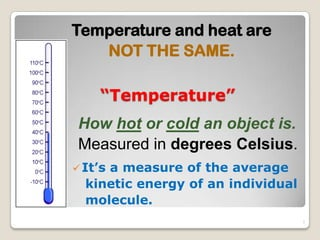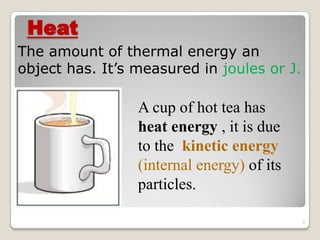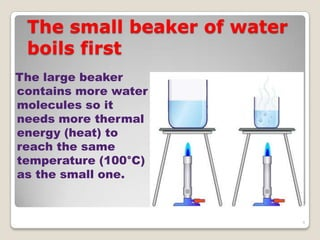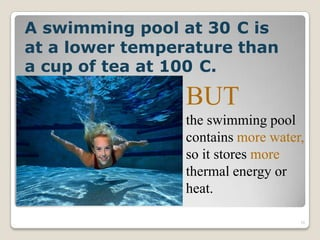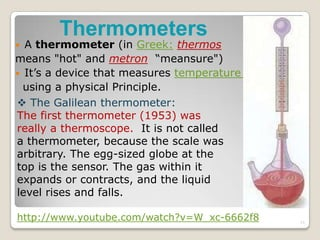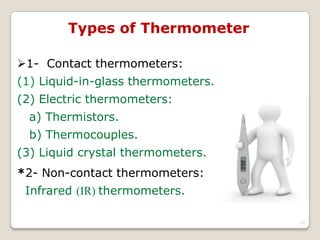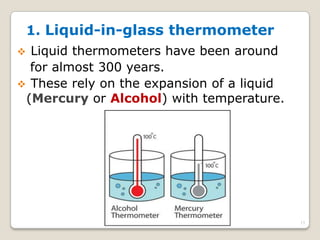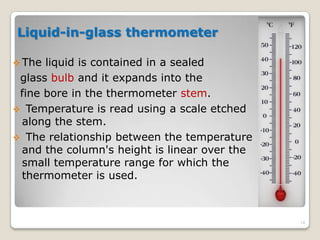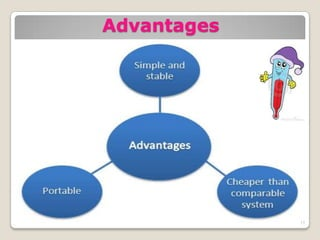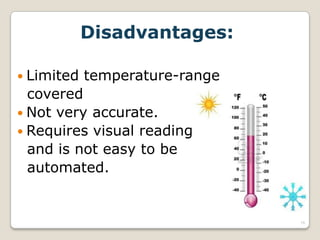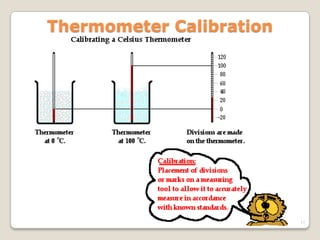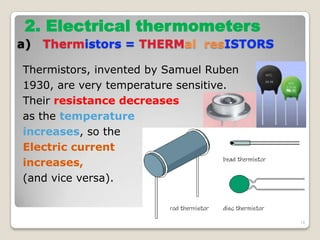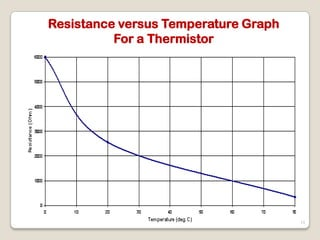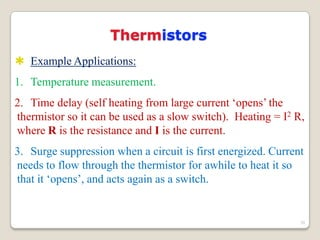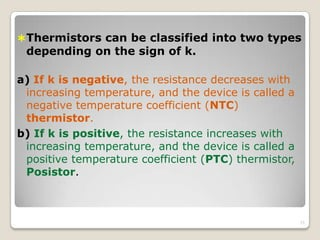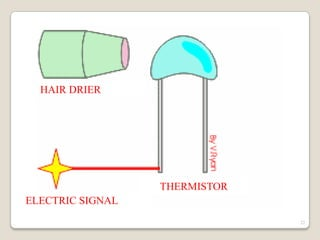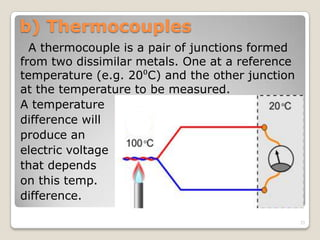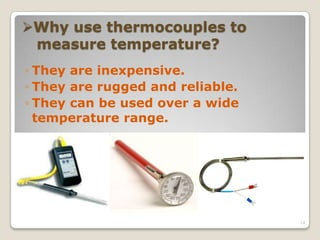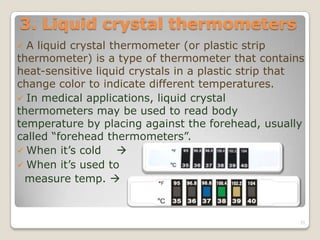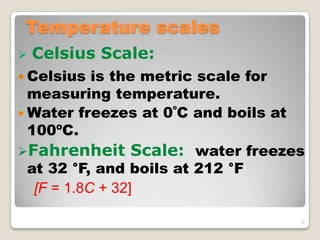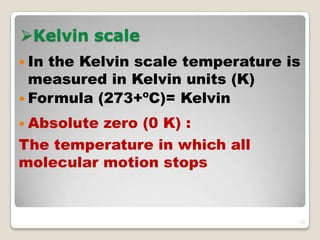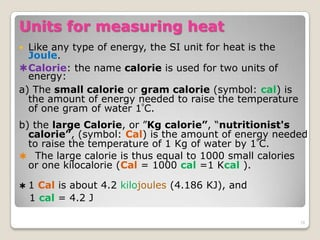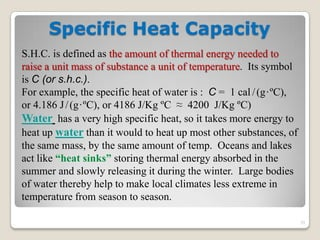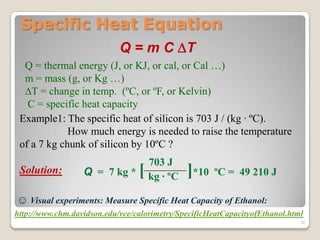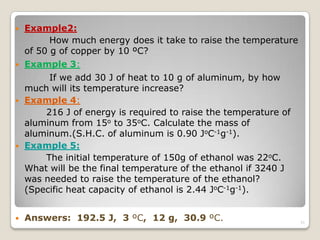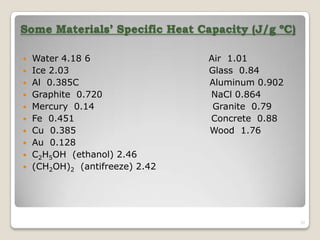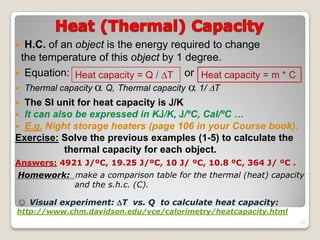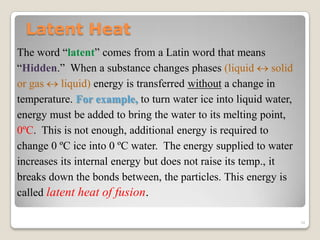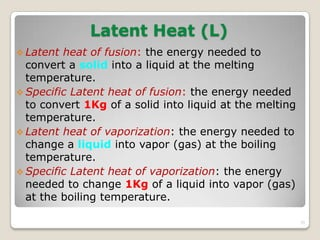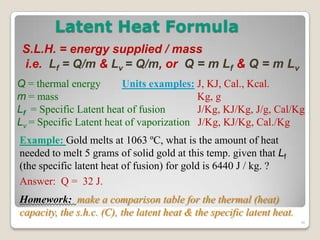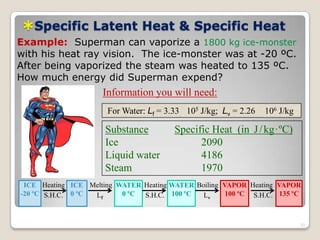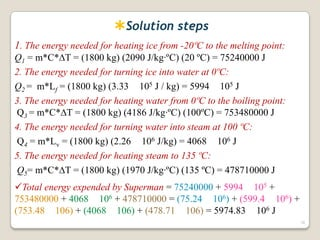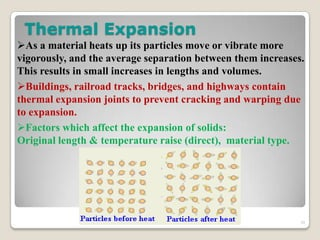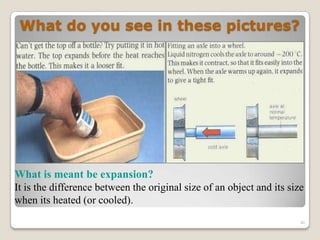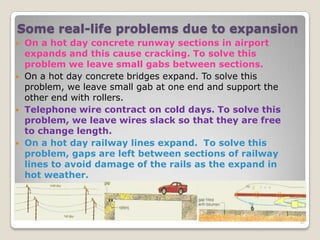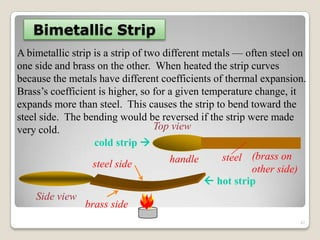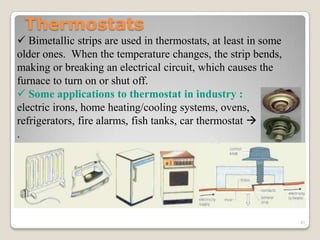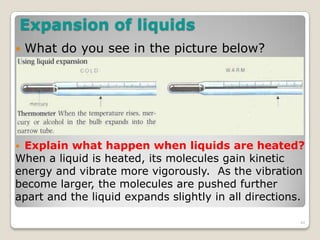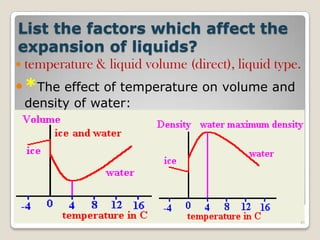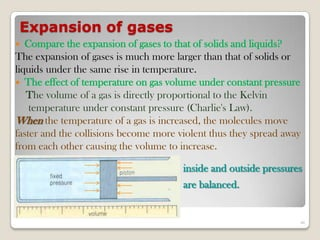1 de 46
Anúncio

### Capter 10

1. Thermal Energy  A burning candle 1
2.  A measure of the average kinetic energy of molecule 2
3. Why is it colder in the night than in the day?  The sun is the greatest heat source.  As the sun comes up, it warms earth.  As the sun goes down, the heat is taken away and it cools off. 3
4. What will happen to this cold snowman throughout the day as the sun warms it up? 4
5. What would happen to the temperature of the boiling water in this kettle if I added ice cubes? 5
6. How is the change in temperature of the snowman and the boiling water related?  The temperature of both the snowman and the boiling water changed to a temperature that was not really cold or really hot, but rather somewhere in between. 6
7. “Temperature” How hot or cold an object is. Measured in degrees Celsius. Temperature and heat are NOT THE SAME. It’s a measure of the average kinetic energy of an individual molecule. 7
8. Heat The amount of thermal energy an object has. It’s measured in joules or J. A cup of hot tea has heat energy , it is due to the kinetic energy (internal energy) of its particles. 8
9. The small beaker of water boils first The large beaker contains more water molecules so it needs more thermal energy (heat) to reach the same temperature (100°C) as the small one. 9
10. A swimming pool at 30 C is at a lower temperature than a cup of tea at 100 C. BUT the swimming pool contains more water, so it stores more thermal energy or heat. 10
11. Thermometers  A thermometer (in Greek: thermos means "hot" and metron “meansure")  It’s a device that measures temperature using a physical Principle.  The Galilean thermometer: The first thermometer (1953) was really a thermoscope. It is not called a thermometer, because the scale was arbitrary. The egg-sized globe at the top is the sensor. The gas within it expands or contracts, and the liquid level rises and falls. http://www.youtube.com/watch?v=W_xc-6662f8 11
12. Types of Thermometer 1- Contact thermometers: (1) Liquid-in-glass thermometers. (2) Electric thermometers: a) Thermistors. b) Thermocouples. (3) Liquid crystal thermometers. *2- Non-contact thermometers: Infrared (IR) thermometers. 12
13. 1. Liquid-in-glass thermometer  Liquid thermometers have been around for almost 300 years.  These rely on the expansion of a liquid (Mercury or Alcohol) with temperature. 13
14. Liquid-in-glass thermometer  The liquid is contained in a sealed glass bulb and it expands into the fine bore in the thermometer stem.  Temperature is read using a scale etched along the stem.  The relationship between the temperature and the column's height is linear over the small temperature range for which the thermometer is used. 14
16. Disadvantages:  Limited temperature-range covered  Not very accurate.  Requires visual reading and is not easy to be automated. 16
17. Thermometer Calibration 17
18. Thermistors, invented by Samuel Ruben 1930, are very temperature sensitive. Their resistance decreases as the temperature increases, so the Electric current increases, (and vice versa). 2. Electrical thermometers a) Thermistors = THERMal resISTORS 18
19. Resistance versus Temperature Graph For a Thermistor 19
20. Thermistors  Example Applications: 1. Temperature measurement. 2. Time delay (self heating from large current „opens‟ the thermistor so it can be used as a slow switch). Heating = I2 R, where R is the resistance and I is the current. 3. Surge suppression when a circuit is first energized. Current needs to flow through the thermistor for awhile to heat it so that it „opens‟, and acts again as a switch. 20
21. Thermistors can be classified into two types depending on the sign of k. a) If k is negative, the resistance decreases with increasing temperature, and the device is called a negative temperature coefficient (NTC) thermistor. b) If k is positive, the resistance increases with increasing temperature, and the device is called a positive temperature coefficient (PTC) thermistor, Posistor. 21
22. ELECTRIC SIGNAL HAIR DRIER THERMISTOR 22
23. b) Thermocouples A thermocouple is a pair of junctions formed from two dissimilar metals. One at a reference temperature (e.g. 20⁰C) and the other junction at the temperature to be measured. A temperature difference will produce an electric voltage that depends on this temp. difference. 23
24. Why use thermocouples to measure temperature? ◦ They are inexpensive. ◦ They are rugged and reliable. ◦ They can be used over a wide temperature range. 24
25. 3. Liquid crystal thermometers  A liquid crystal thermometer (or plastic strip thermometer) is a type of thermometer that contains heat-sensitive liquid crystals in a plastic strip that change color to indicate different temperatures.  In medical applications, liquid crystal thermometers may be used to read body temperature by placing against the forehead, usually called “forehead thermometers”.  When it’s cold   When it’s used to measure temp.  25
26. Temperature scales  Celsius Scale:  Celsius is the metric scale for measuring temperature.  Water freezes at 0ºC and boils at 100ºC. Fahrenheit Scale: water freezes at 32 °F, and boils at 212 °F [F = 1.8C + 32] 26
27. Kelvin scale  In the Kelvin scale temperature is measured in Kelvin units (K)  Formula (273+ºC)= Kelvin  Absolute zero (0 K) : The temperature in which all molecular motion stops 27
28. Units for measuring heat  Like any type of energy, the SI unit for heat is the Joule. Calorie: the name calorie is used for two units of energy: a) The small calorie or gram calorie (symbol: cal) is the amount of energy needed to raise the temperature of one gram of water 1⁰C. b) the large Calorie, or ”Kg calorie”, “nutritionist's calorie”, (symbol: Cal) is the amount of energy needed to raise the temperature of 1 Kg of water by 1⁰C.  The large calorie is thus equal to 1000 small calories or one kilocalorie (Cal = 1000 cal =1 Kcal ).  1 Cal is about 4.2 kilojoules (4.186 KJ), and 1 cal = 4.2 J 28
29. Specific Heat Capacity S.H.C. is defined as the amount of thermal energy needed to raise a unit mass of substance a unit of temperature. Its symbol is C (or s.h.c.). For example, the specific heat of water is : C = 1 cal /(g·ºC), or 4.186 J/(g·ºC), or 4186 J/Kg ºC ≈ 4200 J/Kg ºC) Water has a very high specific heat, so it takes more energy to heat up water than it would to heat up most other substances, of the same mass, by the same amount of temp. Oceans and lakes act like “heat sinks” storing thermal energy absorbed in the summer and slowly releasing it during the winter. Large bodies of water thereby help to make local climates less extreme in temperature from season to season. 29
30. Specific Heat Equation Q = m C T Q = thermal energy (J, or KJ, or cal, or Cal …) m = mass (g, or Kg …) T = change in temp. (ºC, or ºF, or Kelvin) C = specific heat capacity Example1: The specific heat of silicon is 703 J / (kg · ºC). How much energy is needed to raise the temperature of a 7 kg chunk of silicon by 10ºC ? Solution: 703 J kg · ºCQ = 7 kg * [ ]*10 ºC = 49 210 J ☺ Visual experiments: Measure Specific Heat Capacity of Ethanol: http://www.chm.davidson.edu/vce/calorimetry/SpecificHeatCapacityofEthanol.html 30
31.  Example2: How much energy does it take to raise the temperature of 50 g of copper by 10 ºC?  Example 3: If we add 30 J of heat to 10 g of aluminum, by how much will its temperature increase?  Example 4: 216 J of energy is required to raise the temperature of aluminum from 15o to 35oC. Calculate the mass of aluminum.(S.H.C. of aluminum is 0.90 JoC-1g-1).  Example 5: The initial temperature of 150g of ethanol was 22oC. What will be the final temperature of the ethanol if 3240 J was needed to raise the temperature of the ethanol? (Specific heat capacity of ethanol is 2.44 JoC-1g-1).  Answers: 192.5 J, 3 ºC, 12 g, 30.9 ºC. 31
32. Some Materials’ Specific Heat Capacity (J/g ºC)  Water 4.18 6 Air 1.01  Ice 2.03 Glass 0.84  Al 0.385C Aluminum 0.902  Graphite 0.720 NaCl 0.864  Mercury 0.14 Granite 0.79  Fe 0.451 Concrete 0.88  Cu 0.385 Wood 1.76  Au 0.128  C2H5OH (ethanol) 2.46  (CH2OH)2 (antifreeze) 2.42 32
33.  H.C. of an object is the energy required to change the temperature of this object by 1 degree.  Equation: or  Thermal capacity  Q, Thermal capacity  1/ T  The SI unit for heat capacity is J/K  It can also be expressed in KJ/K, J/ºC, Cal/ºC …  E.g. Night storage heaters (page 106 in your Course book). Exercise: Solve the previous examples (1-5) to calculate the thermal capacity for each object. Answers: 4921 J/ºC, 19.25 J/ºC, 10 J/ ºC, 10.8 ºC, 364 J/ ºC . Homework: make a comparison table for the thermal (heat) capacity and the s.h.c. (C). Heat capacity = Q / T Heat capacity = m * C ☺ Visual experiment: T vs. Q to calculate heat capacity: http://www.chm.davidson.edu/vce/calorimetry/heatcapacity.html 33
34. Latent Heat The word “latent” comes from a Latin word that means “Hidden.” When a substance changes phases (liquid  solid or gas  liquid) energy is transferred without a change in temperature. For example, to turn water ice into liquid water, energy must be added to bring the water to its melting point, 0ºC. This is not enough, additional energy is required to change 0 ºC ice into 0 ºC water. The energy supplied to water increases its internal energy but does not raise its temp., it breaks down the bonds between, the particles. This energy is called latent heat of fusion. 34
35. Latent Heat (L)  Latent heat of fusion: the energy needed to convert a solid into a liquid at the melting temperature.  Specific Latent heat of fusion: the energy needed to convert 1Kg of a solid into liquid at the melting temperature.  Latent heat of vaporization: the energy needed to change a liquid into vapor (gas) at the boiling temperature.  Specific Latent heat of vaporization: the energy needed to change 1Kg of a liquid into vapor (gas) at the boiling temperature. 35
36. Latent Heat Formula S.L.H. = energy supplied / mass i.e. Lf = Q/m & Lv = Q/m, or Q = m Lf & Q = m Lv Q = thermal energy Units examples: J, KJ, Cal., Kcal. m = mass Kg, g Lf = Specific Latent heat of fusion J/Kg, KJ/Kg, J/g, Cal/Kg Lv = Specific Latent heat of vaporization J/Kg, KJ/Kg, Cal./Kg Example: Gold melts at 1063 ºC, what is the amount of heat needed to melt 5 grams of solid gold at this temp. given that Lf (the specific latent heat of fusion) for gold is 6440 J / kg. ? Answer: Q = 32 J. Homework: make a comparison table for the thermal (heat) capacity, the s.h.c. (C), the latent heat & the specific latent heat. 36
37. Specific Latent Heat & Specific Heat Substance Specific Heat (in J/kg·ºC) Ice 2090 Liquid water 4186 Steam 1970 Example: Superman can vaporize a 1800 kg ice-monster with his heat ray vision. The ice-monster was at -20 ºC. After being vaporized the steam was heated to 135 ºC. How much energy did Superman expend? For Water: Lf = 3.33 105 J/kg; Lv = 2.26 106 J/kg Information you will need: ICE -20 ºC ICE 0 ºC WATER 0 ºC VAPOR 100 ºC WATER 100 ºC VAPOR 135 ºC Heating Melting Heating Boiling Heating S.H.C. Lf S.H.C. Lv S.H.C. 37
38. Solution steps 1. The energy needed for heating ice from -20ºC to the melting point: Q1 = m*C*T = (1800 kg) (2090 J/kg·ºC) (20 ºC) = 75240000 J 2. The energy needed for turning ice into water at 0ºC: Q2 = m*Lf = (1800 kg) (3.33 105 J / kg) = 5994 105 J 3. The energy needed for heating water from 0ºC to the boiling point: Q3 = m*C*T = (1800 kg) (4186 J/kg·ºC) (100ºC) = 753480000 J 4. The energy needed for turning water into steam at 100 ºC: Q4 = m*Lv = (1800 kg) (2.26 106 J/kg) = 4068 106 J 5. The energy needed for heating steam to 135 ºC: Q5= m*C*T = (1800 kg) (1970 J/kg·ºC) (135 ºC) = 478710000 J Total energy expended by Superman = 75240000 + 5994 105 + 753480000 + 4068 106 + 478710000 = (75.24 106) + (599.4 106) + (753.48 106) + (4068 106) + (478.71 106) = 5974.83 106 J 38
39. Thermal Expansion As a material heats up its particles move or vibrate more vigorously, and the average separation between them increases. This results in small increases in lengths and volumes. Buildings, railroad tracks, bridges, and highways contain thermal expansion joints to prevent cracking and warping due to expansion. Factors which affect the expansion of solids: Original length & temperature raise (direct), material type. 39
40. What do you see in these pictures? What is meant be expansion? It is the difference between the original size of an object and its size when its heated (or cooled). 40
41. Some real-life problems due to expansion  On a hot day concrete runway sections in airport expands and this cause cracking. To solve this problem we leave small gabs between sections.  On a hot day concrete bridges expand. To solve this problem, we leave small gab at one end and support the other end with rollers.  Telephone wire contract on cold days. To solve this problem, we leave wires slack so that they are free to change length.  On a hot day railway lines expand. To solve this problem, gaps are left between sections of railway lines to avoid damage of the rails as the expand in hot weather. 41
42. Bimetallic Strip A bimetallic strip is a strip of two different metals — often steel on one side and brass on the other. When heated the strip curves because the metals have different coefficients of thermal expansion. Brass‟s coefficient is higher, so for a given temperature change, it expands more than steel. This causes the strip to bend toward the steel side. The bending would be reversed if the strip were made very cold. cold strip   hot strip handle steel (brass on other side) Top view steel side brass side Side view 42
43. Thermostats  Bimetallic strips are used in thermostats, at least in some older ones. When the temperature changes, the strip bends, making or breaking an electrical circuit, which causes the furnace to turn on or shut off.  Some applications to thermostat in industry : electric irons, home heating/cooling systems, ovens, refrigerators, fire alarms, fish tanks, car thermostat  . 43
44. Expansion of liquids  What do you see in the picture below?  Explain what happen when liquids are heated? When a liquid is heated, its molecules gain kinetic energy and vibrate more vigorously. As the vibration become larger, the molecules are pushed further apart and the liquid expands slightly in all directions. 44
45. List the factors which affect the expansion of liquids?  temperature & liquid volume (direct), liquid type. *The effect of temperature on volume and density of water: 45
46. Expansion of gases  Compare the expansion of gases to that of solids and liquids? The expansion of gases is much more larger than that of solids or liquids under the same rise in temperature.  The effect of temperature on gas volume under constant pressure The volume of a gas is directly proportional to the Kelvin temperature under constant pressure (Charlie's Law). When the temperature of a gas is increased, the molecules move faster and the collisions become more violent thus they spread away from each other causing the volume to increase. inside and outside pressures are balanced. 46
Anúncio